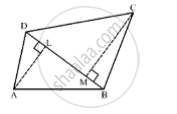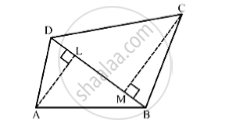# In the Given Figure Abcd is Quadrilateral in Which Diagonal Bd = 24 Cm, Al ⊥ Bd and Cm ⊥ Bd Such that Al = 9cm and Cm = 12 Cm. Calculate the Area of the Quadrilateral. - Mathematics

In the given figure ABCD is quadrilateral in which diagonal BD = 24 cm, AL ⊥ BD  and CM ⊥ BD  such that AL = 9cm and CM = 12 cm. Calculate the area of the quadrilateral.#### SolutionWe have,

BD=24 cm AL=9 cm CM=12 cm AL⊥ BD and CM ⊥BD

Area of the quadrilateral = ar (Δ ABD)+ar (Δ BCD)

= 1/2xx BDxxAL+1/2 xxBDxxCM

=1/2xx24xx9+1/2xx24xx12

=108+144

=252 cm^2

So, the area of the quadrilateral ABCD is 252cm^2

Concept: Area of Circle
Is there an error in this question or solution?

#### APPEARS IN

RS Aggarwal Secondary School Class 10 Maths
Chapter 17 Perimeter and Areas of Plane Figures
Q 24